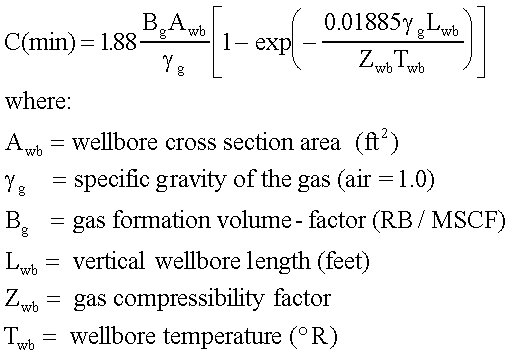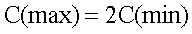## ESTIMATE THE STORAGE-COEFFICIENT

The next piece of information required is an estimate of the possible range of the well-bore storage-coefficient. This coefficient represents the volume of fluid that enters the well-bore per unit-change in the bottom-hole pressure. Because this is a well-bore coefficient, it can be estimated strictly from the well-bore geometry and the gas in the well-bore.

FIRST: calculate the MINIMUM storage coefficient, C(min), from a formula based on the "barometric equation". This value corresponds to a stagnant column of gas at a uniform temperature.SECOND: calculate the MAXIMUM storage coefficient, C(max) as:Calculate C(min) and C(max) and write down the values you obtain; we need them later!

Q: Did you calculate C(min) and C(max) and write down the values?